# waynehu

## Professor, Department of Astronomy and Astrophysics University of Chicago

Group Contact CV SnapShots

## Electric and Magnetic Modes

Any polarization pattern on the sky can be separated into ``electric'' (E) and ``magnetic'' (B) components. This decomposition is useful both observationally and theoretically, as we will discuss below. There are two equivalent ways of viewing the modes that reflect their global and local properties respectively. The nomenclature reflects the global property. Like multipole radiation, the harmonics of an E-mode have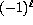parity on the sphere, whereas those of a B-mode have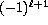parity. Under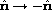, the E-mode thus remains unchanged for even, whereas the B-mode changes sign as illustrated for the simplest case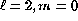in Fig. 9 (recall that a rotation by 90represents a change in sign). Note that the E and B multipole patterns are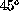rotations of each other, i.e.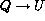and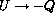. Since this parity property is obviously rotationally invariant, it will survive integration over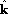.

Fig. 9: The electric (E) and magnetic (B) modes are distinguished by their behavior under a parity transformation n → -n. E modes have (-1)l parity and B modes have (-1)l+1, here (l=2, m=0), even and odd respectively. The local distinction between the two is that the polarization direction is aligned with the principal axes of the polarization amplitude for E and crossed (45 degrees) for B. Dotted lines represent a sign reversal in the polarization.

The local view of E and B-modes involves the second derivatives of the polarization amplitude (second derivatives because polarization is a tensor or spin-2 object). In much the same way that the distinction between electric and magnetic fields in electromagnetism involves vanishing of gradients or curls (i.e. first derivatives) for the polarization there are conditions on the second (covariant) derivatives of Q and U. For an E-mode, the difference in second (covariant) derivatives of U along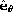and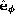vanishes as does that for Q along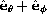and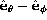. For a B-mode, Q and U are interchanged. Recalling that a Q-field points in theordirection and a U-field in the crossed direction, we see that the Hessian or curvature matrix of the polarization amplitude has principle axes in the same sense as the polarization for E and 45crossed with it for B (see Fig. 9). Stated another way, near a maximum of the polarization (where the first derivative vanishes) the direction of greatest change in the polarization is parallel/perpendicular and atdegrees to the polarization in the two cases.

The distinction is best illustrated with examples. Take the simplest case of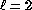, m=0 where the E-mode is a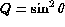field and the B-mode is a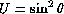field (see Fig. 4). In both cases, the major axis of the curvature lies in thedirection. For the E-mode, this is in the same sense; for the B-mode it is crossed with the polarization direction. The same holds true for the m=1,2 modes as can be seen by inspection of Fig. 6 and 8.